GET THE APP

Dersleri yüzünden oldukça stresli bir ruh haline sikiş hikayeleri bürünüp özel matematik dersinden önce rahatlayabilmek için amatör pornolar kendisini yatak odasına kapatan genç adam telefonundan porno resimleri açtığı porno filmini keyifle seyir ederek yatağını mobil porno okşar ruh dinlendirici olduğunu iddia ettikleri özel sex resim bir masaj salonunda çalışan genç masör hem sağlık hem de huzur sikiş için gelip masaj yaptıracak olan kadını gördüğünde porn nutku tutulur tüm gün boyu seksi lezbiyenleri sikiş dikizleyerek onları en savunmasız anlarında fotoğraflayan azılı erkek lavaboya geçerek fotoğraflara bakıp koca yarağını keyifle okşamaya başlar
Critical Analysis of Two Respirometric Methods for Solid Substrates Based On Continuous and Semi-Continuous Aeration | OMICS International

Like us on:

# Critical Analysis of Two Respirometric Methods for Solid Substrates Based On Continuous and Semi-Continuous Aeration

 Rada EC*, Ragazzi M and Venturi M Department of Civil and Environmental Engineering, University of Trento, Via Mesiano 77, I-38050 Trento, Italy Corresponding Author : Rada EC Department of Civil and Environmental Engineering University of Trento, Via Mesiano 77 I-38050 Trento, Italy E-mail: [email protected] Received: August 01, 2012; Accepted: October 10, 2012; Published: October 27, 2012 Citation: Rada EC, Ragazzi M, Venturi M (2012) Critical Analysis of Two Respirometric Methods for Solid Substrates Based On Continuous and Semi- Continuous Aeration. J Bioremed Biodeg 3:170. doi:10.4172/2155-6199.1000170 Copyright: © 2012 Rada EC, et al. This is an open-a ccess article distributed under the terms of the Creative Commons Attribution License, which permits unrestricted use, distribution, and reproduction in any medium, provided the original author and source are credited. Related article at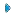PubmedScholar Google

Visit for more related articles at Journal of Bioremediation & Biodegradation

#### Abstract

In the sector of respirometry applied to solid waste many tools are available. Moreover, methods for assessing the oxygen consumption can be significantly different. In Italy, the sector is characterized by the adoption of dynamic approaches that are preferred to the static ones. In this paper, a comparison between the two respirometers more adopted in Italy is presented. The first one is the Costech respirometer, with continuous aeration and without temperature control. The second one is the AIR-nl respirometer, with temperature control and a semicontinuous aeration based on the principle of avoiding limiting conditions of oxygen. Results were obtained during an experimental research that involved on one hand the construction of an analytical model for evaluating the Respirometric Index (RI) for various substrates and on the other, the critical analysis of the obtained measurements. In this paper, the relationship between the output of tests conducted in parallel with two different instruments on the same samples is analyzed. Some differences between the results of the measurements and obtainable parameters (RI24) are underlined. Considerations on reference moisture, temperature role, and respirometric index calculation method allow the understanding of the characteristics of the two methods in more detail.

Keywords
Moisture; Respirometry; Respirometric index; Temperature
Introduction
Since 1999, with the Directive 1999/31/CE (Italian Council Directive 1999), the European Union (EU) has imposed limits for waste landfilling (lower heating value and biodegradable matter targets) and also technical requirements for waste treatment plants in order to decrease their negative effects on the environment . To this concern, one of the most important parameters is the respiration activity.
In Italy, with the transposition of the EU technical norm CEN/TR 15590 (Italian National Standard Body 2007) into the decree 205/2010, this parameter is also requested for the output of the mechanicalbiological plants aimed to energy recovery through solid recovered fuel (SRF) generation [2,3].
The biomass respirability or the Respirometric Index (RI) is the amount of oxygen consumed from the microorganisms. Generally, oxygen consumption is referred to as an amount of volatile solids (VS, also called Organic Matter, OM) during a fixed time . The respirometric tests based on oxygen consumption can be classified in static and dynamic methods depending on the oxygen aeration type .
• Absent aeration (static): O2 consumption is usually measured via pressure differences or via direct measurements of the O2 content in air or liquid medium [6-8]; in Italy static respirometry is established by the Italian Organization for Standardization with the norm UNI 10780 (Italian Standard Body 1998) .
• Continuous aeration (dynamic): it requires the precise measurement of the air flow rate and the O2 content at the inlet and outlet of the test devices [10,11]; in Italy, dynamic respirometry is established by the Italian Organization for Standardization with the norm UNI/TS 11184 (Italian Standard Body 2006) .
The static method limits oxygen circulation and dispersion in the substrate slowing the degradation processes of the organic matter. Moreover, it does not allow the removal of the exhausted air from the instrumentation. The pH decrease and the toxicity phenomena related to CO2 or other fermentation gas accumulation cause the slowing of the biological activity. For those reasons, the static method underestimates the oxygen consumption. On the contrary, in the dynamic method, the measurement of the oxygen consumption is made taking into account the continuous aeration.
Additionally, differences related to the respirometric index methods concern the time of reference for the assessment of its value. In Austria and in Germany, for instance, the reference parameter is the AT4 which represents the respirometric activity calculated on four days and referred to dry matter (DM). To this concern, the limit for waste landfilling is respectively 7 mgO2 gDM-1 and 5 mgO2 gDM-1 .
In general, the dynamic method to which the Italian regulations refer is the Costech one, developed by DiProVe . Parallel to this approach, in the University of Trento another system for measuring the dynamic respirability of a substrate, the AIR-nl Respirometer, has been developed [14,15].
Considering the significant differences of approach of the two instruments and more in general the fragmentation of the national regulations on respirometry, the present paper was developed with the aim of contributing to a correct adoption of both of the devices, in order to obtain only one respirability value from both of them. To this concern, in this paper, the relationship between the output of tests conducted in parallel with the two instruments on the same samples was analyzed. Differences between the results of the measurements and the obtainable parameters (RI24) were underlined too. Some considerations on reference moisture for sample pre-treatment, test temperature, respirometric index calculation criteria were developed for understanding more in details the potential of the two methods.
Materials and Methods
The main characteristics of the two methods are described below. The Costech respirometer is an adiabatic reactor with an available volume of 30 liters. This instrument has a flux regulator, a flow meter, an air humidification system, an oxygen meter at the inlet and at the outlet, temperature probes for internal and external measurements. An integrated system registers in continuous oxygen concentration, temperature and air flow. A system for leachate collection completes the device in order to avoid troubles with condensation phenomena.
According to the method, 10-13 kg of the wet samples must be used for the tests . Samples must be optimized in terms of moisture content (a standard of 75% of water content must be complied with). The obtained sample must be placed in the respirometer and continuously aerated using flow values able to guarantee an outlet O2 concentration higher than 14% (v/v). This value is compulsory for keeping aerobic conditions. Temperature is not kept steady. An hourly dynamic respirometric index is assessed in mgO2 kg-1vs h-1 and comes from the air flow-rate, form the difference in the O2 concentration in the inlet and outlet air flows and from the initial VS content.
The AIR-nl respirometer is an adiabatic reactor having one probe for temperature and one probe for oxygen measurements [14,15]. The reactor is kept at the chosen temperature by a thermostatic bath (30°C). The system of aeration and analysis is composed by a double pneumatic circuit: in the first one, at low flow, the oxygen concentration is measured in continuous, checking that its value is always higher than 18.5% (v/v); indeed the second one, at higher flow, guarantees the re-oxygenation by a continuous flow of air through the sample. This last circuit has two electro-valves that allow the control of O2 concentration opening or closing the circuit. An hourly dynamic respirometric index is assessed in mgO2 kg-1 vs h-1. Moisture in the sample is corrected to 55% before the test, and one kg of sample is used for the tests.
In order to make a comparison between the two instruments, some experimental data from the runs developed at the Trento University, regarding the oxygen consumption of compost samples were originally used in this paper. Two sets of data were used. The first one regarded the calculated values of RI24 and the reference average temperature of the tests. The second one consisted of detailed data concerning oxygen consumption for additional runs. Those data came from tests developed in parallel on the same substrate, with both the respirometric instruments working in parallel. The two equipments are presented in figure 1.
Data collected from the two dynamic respirometers regarding the oxygen consumption were disposed along a negative slope. The average gradient of each series of data linearly decreasing, referred to the average time, gave the value of the specific respirometric activity (mgO2 h-1), that was referred to the volatile solids available in the system. The respirometric index RI was then calculated by the following expression (Equation 1):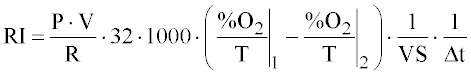(1)
Where,
P=pressure [atm] assumed equal to the atmospheric one;
V=free volume [liter];
T=temperature [K];
32=oxygen molecular weight [g];
VS=volatile solids [kg];
Δt =time interval [h];
R=0.0821 [l.atm.K-1.mol-1] constant of the perfect gas;
1 and 2=points between the ones the slope is calculated.
The respirometric index dynamics in the time was then represented by a continuous curve and was calculated through a mass balance using the slope.
Regarding data recorded with the Costech Respirometer, it was needed to report oxygen consumption to the reference temperature of 30°C, in order to compare the values with the ones from the Air-nl Respirometer. The literature proposes an expression (Equation 2) valid in the range between 20 and 40°C :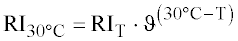(2)
Where,
T=instantaneous temperature at which the measure is done (with the temperature probe placed into the substrate)
ϑ=reference constant, equal to 1.08.
From the modified values, for each time step, the area under the respirometric curve was assessed and from that the sum until a stated instant. In practice, it was possible to assess the cumulative oxygen consumption referred to a fixed time.
After that, the RI24 calculation was made using the following expression (Equation 3):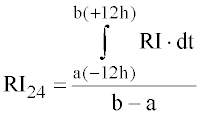(3)
where referring to a fixed time, a and b indicate respectively the initial time at -12 hours and the final time at +12 hours.
The RI24 values were obtained associating at each time the corresponding value of the integral average of RI in an interval of 24 hours centered on the considered time. According to the method, the RI value to be associated to each run is the highest of the RI24 values. The run can be stopped when the slope of the integral curve of RI24, determined on a period longer than 12 hours, is negative.
According to the AIR-nl method, the acceptability of the tests conducted with that respirometer depends on the behavior of the temperature value during the overall run; if temperature varies by more than ± 2°C, the measurement should be repeated. Indeed, AIR-nl respirometer works at steady temperature.
For the comparison between the two devices, it was needed to modify data taken from AIR-nl to the average temperature registered by Costech, for the same analyzed sample. When the average temperature in a Costech test was higher than 40°C, the resulting respirometric value had to be considered not acceptable for a comparison after temperature correction. The reason is related to Equation 2 that is suitable until 40°C.
The used samples were taken from different composting plants. For a better analysis of the stabilization, development sample ages were selected in the range of 7-45 days.
Additional data were generated from three samples analyzed in parallel recording the cumulative O2 consumption during the same period (66 hours).
Results
The available measurements and the calculation results are presented in Table 1 (first series of data). It can be underlined that RI24 values measured with Costech are always lower than the ones from AIR-nl after temperature correction. This aspect clearly contradicts the statement that defines the system AIR-nl as a tool that is not completely dynamic, involving “an underestimation of oxygen consumption” . An important parameter is the moisture percentage. Some studies showed that the increased biological activity, underlined as maximum oxygen consumption, is found in correspondence with moisture values that can range from 50% to 70% . From the moisture point of view, samples carried out with the AIR-nl Respirometer has the advantage to be corrected to an optimal reference value of 55%, (as the method requires) providing an acceptability range of 2.5 percentage points . The reference moisture value for the Costech method is equal to 75%, a value that cannot be considered optimal in this sense.
From data reported in table 1 that were considered acceptable, a graph about RI24, measured with the AIR-nl and Costech respirometers, was obtained and is presented in figure 2. As it can be seen, the obtained correlation has a linear fitting, with a correlation coefficient equal to 0.9640. Taking into account that the same samples were used, the experimental values obtained with the Costech device were on average lower than those resulted with the AIR-nl (indeed the slope resulted 0.7).
For a deeper comparative analysis, results of additional tests performed in parallel with both respirometers are shown in table 2 and figure 3. It can be noticed that the linear relationship between the two measurements is very high (there is a correlation coefficient of about 0.9940), and this analysis provides further support to the analytical method developed in a previous study . Form figure 2 it can be noticed that there is no difference between the two respirometers concerning the ratio between cumulative oxygen and RI24.
The numerical interpolation relation showed a slope very similar to the one theoretically assessed from the organic matter composition in Venturi et al. . The slope of the previous experimental work was 0.01432; the slope of the straight line for the present research shown in figure 4 is equal to 0.01425. Considerations about the intercept of the straight interpolation were made: this value resulted very small (especially when compared to typical respirometric values of 1,000÷3,000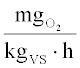and because of that it was chosen to bring it to zero in order to obtain a new relation.
It can be seen that the coefficient of determination does not suffer a significant decrease reaching the value 0.9639. Using the derived relationship (Equation 4) and recalling that the proposed limit of acceptability for admission to landfills by the Costech method showed a value of 1,000, it is possible to obtain the reference value for the
AIR-nl respirometer by reversing the relationship. The corresponding value is equal to 1411.19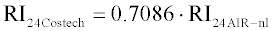(4)
By combining the two relations (Equation 2 and Equation 4), the following expression (Equation 5) was obtained: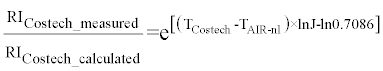(5)
Where,
RICostech_measured is the respirometric measurement presented in table 1;
RICostech_calculated is obtained after the measurement with AIR-nl respirometer, referring to Costech;
TCostech is the average temperature for the Costech respirometric runs;
TAIR-nl is the average temperature for the AIR-nl respirometric runs;
Taking into account the equation 5, in figure 5 values obtained from table 1 were reported. It can be seen that the indicated deviations are not correlated to the difference of average operating temperature of the two respirometers. Data reported in table 1 refer to the output of the software for the overall respirometric curve. Thus, the calculation of RI24 with the same methodology for both instruments was not possible. Indeed, for the Costech respirometer the RI24 was calculated using the following expression (Equation 6):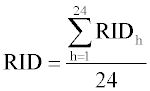(6)
and for AIR-nl respirometer, the RI24 was calculated using the following expression (Equation 7):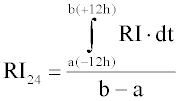(7)
where a and b indicate the initial time at -12 hours, respectively, the final time at +12 hours, with peak time reference.
In order to evaluate the magnitude error that was made, it was decided to use oxygen consumption for calculating the RI24 from Costech measurements, with the methodology suggested by the expression used to calculate the RI24 for AIR-nl (Equation 7).
The expression (Equation 8) used to determine the error in percentage was: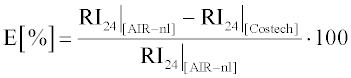(8)
where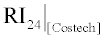and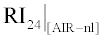indicate the values obtained for RI24 with the first and second expression considered by the two methodologies.
For the three data available for this elaboration (Table 2), the obtained values were equal to -0.0529%, -0.0038% and -0.5128%.They can be considered negligible.
In any case, the following analytical method was considered useful for the comparison of the two calculation methods. For this method, three data from the respirometric activity in consequential moments were considered (Figure 6a). Having to calculate, according to the reference methodology of AIR-nl, the integral of the respirometric curve, b1, b2 and b3 will indicate the measured values (which correspond to the “bases” of trapezoids for the area calculation), h1 and h2 will indicate the time interval between the measurements (corresponding to the heights of the two trapezoids) and A1 and A2 the values of the two areas.
The value of A1 and A2 are expressed as presented in equation 9: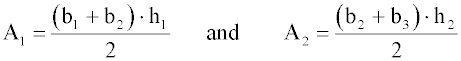(9)
Thus, the RI24 value in the case of only three data may be calculated using the following expression (Equation 10):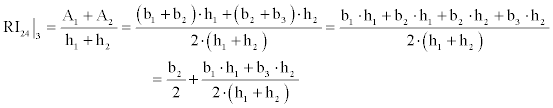(10)
If there is an additional point of measurement (Figure 6b) it is possible to express the area A3 in a similar way and in this case the calculation of RI24 for four data can be expressed as (Equation 11):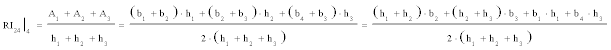(11)
At this point, it is possible to evaluate the RI24 expression (Equation 12) reported to the n-th measure: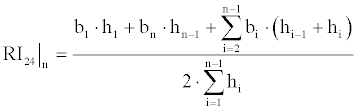(12)
In the case of measurements made with the Costech respirometer, there is a constant interval of time between a measurement and another, equal to 1 hour. If, for simplicity, the same hypothesis is taken also for the AIR-nl, we will have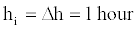. The total time is 24 hours, resulting, therefore, in the presence of 25 measures.
Because of these simplified observations and assumptions, the expression changes, becoming simpler (a change of indices is done for a comparison with the method Costech):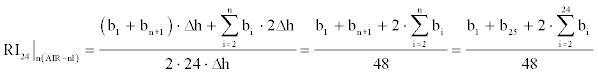(13)
The characteristic relation for Costech (Equation 14) can be expressed as: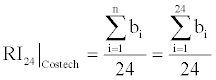(14)
While for the reference method of AIR-nl, 24 areas with 25 measures were used for the calculation (the extremities of analysis interval at -12 hours and +12 hours from the initial peak, are identified), for the method implemented by Costech a simple arithmetic average for 24 hours is done, considering 24 measures. For a comparison between the two methods of calculation, it is possible to calculate the difference between the derived expressions (Equation. 15):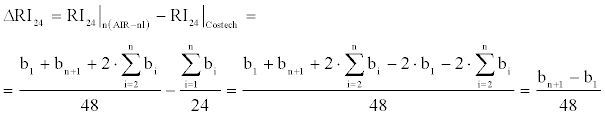(15)
The result can be expressed with a more usual notation (Equation 16):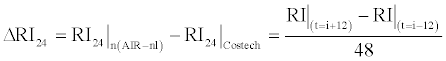(16)
where i refers to the time at which the respirometric peak is reached.
This expression (Equation 16) was applied to the data of the respirometric graphs of the analyzed oxygen consumption. In all the cases, the result was the same with the difference between the two RI24 assessed with the two calculation methods presented in this paper.
Conclusions
The comparison between the two analyzed respirometers (Costech and AIR-nl) pointed out that the development of this kind of instrumentation must be coupled with a careful development of the methodology to be used. Indeed the critical analysis of this approach has demonstrated that some misunderstandings should be solved or corrected.
For instance, the AIR-nl respirometer is considered in some circles a system that underestimate the oxygen consumption. On the contrary, this paper demonstrated that the underestimation is a characteristic of the Costech respirometer.
Another aspect is related to the choice of the moisture of reference (indeed moisture must be corrected before performing the respirometric test); the reference value proposed in the methodology coupled with Costech seems to be too high, resulting out of the range generally considered not limiting.
The role of temperature is another important parameter to be considered: the AIR-nl respirometer is based on the concept of steady temperature during the test (temperature is set according to the proposed method). In the approach proposed by Costech, the temperature is not regulated.
As a consequence of these differences, it is important to develop correlations recognized at international level in order to avoid a non optimized use of those devices which are more and more adopted in the waste and biomass sector.

#### Tables and Figures at a glance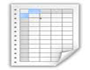Table 1 Table 2

#### Figures at a glance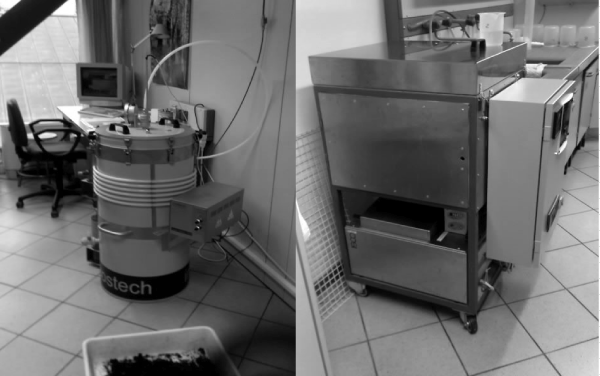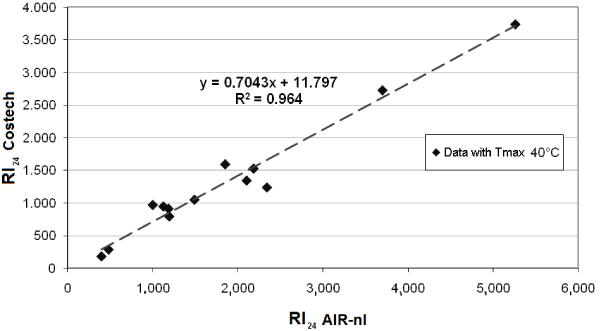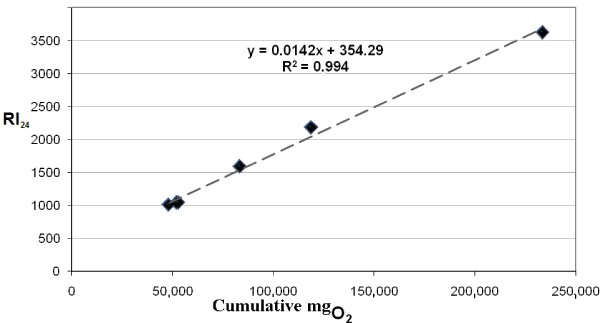Figure 1 Figure 2 Figure 3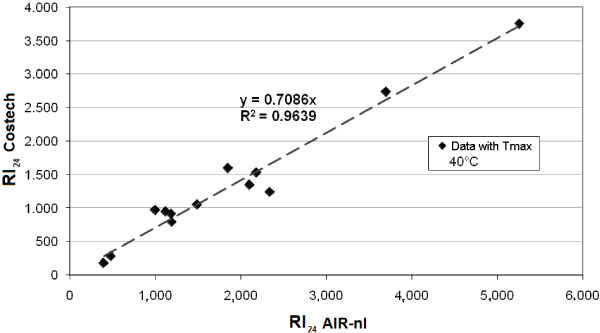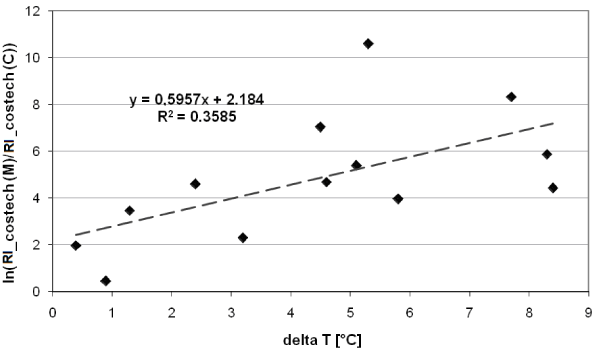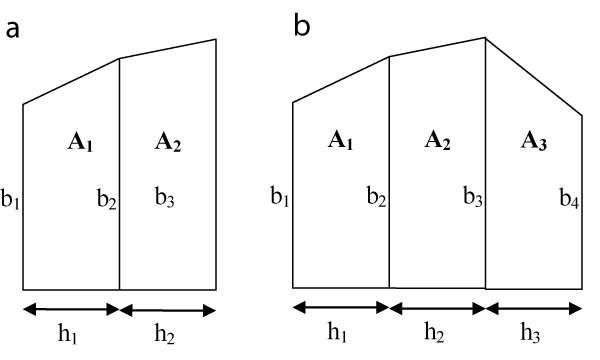Figure 4 Figure 5 Figure 6
Select your language of interest to view the total content in your interested language

### Article Usage

• Total views: 12914
• [From(publication date):
December-2012 - Aug 19, 2022]
• Breakdown by view type
• HTML page views : 8710Can't read the image? click here to refresh